Example Questions

2 Next →

Example Question #11 : How To Find The Slope Of A Line

What is the slope of the line perpendicular to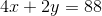?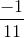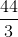Explanation:

To begin, it is easiest to find the slope of a line by putting it into the form.is the slope, so you can immediately find this once you have the format correct.  Thus, solve our equation for: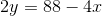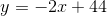Now, recall that perpendicular lines have slopes of opposite sign and reciprocal numerical value.  Thus, if our slope is, its perpendicular paired line will have a slope of.

Example Question #12 : How To Find The Slope Of A Line

What is the slope of the line defined by the equation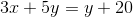?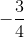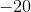Explanation:

The easiest way to find the slope of a line based on its equation is to put it into the form. In this form, you know thatis the slope.

Start with your original equation.

Now, subtractfrom both sides: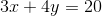Next, subtractfrom both sides: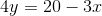Finally, divide by: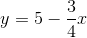This is the same as: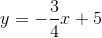Thus, the slope is.

Example Question #13 : How To Find The Slope Of A Line

What is the slope of the line represented by the equation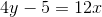?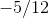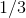Explanation:

The slope of an equation can be calculated by simplifying the equation to the slope-intercept form, where m=slope.

Since, we can solve for y. In shifting the 5 to the other side, we are left with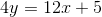.

This can be further simplified to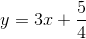, leaving us with theslope intercept form.

In this scenario,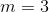, so slope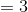.

Example Question #9 : How To Find The Slope Of A Line

Find the slope of the line  6X – 2Y = 14

-6

3

12

-3

3

Explanation:

Put the equation in slope-intercept form:

y = mx + b

-2y = -6x +14

y = 3x – 7

The slope of the line is represented by M; therefore the slope of the line is 3.

2 Next →